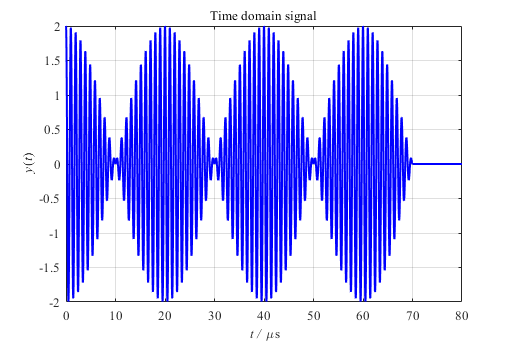## 快速傅里叶变换 FFT

clear;clc
close all
%% FFT
Fs = 100e6;		    % 采样频率 Hz
T = 1/Fs;		    % 采样周期 s
L0 = 1000;                  % 信号长度
L = 1000;                   % 数据长度
t0 = (0:L0-1)*T;            % 信号时间序列
x = cos(2*pi*1e6*t0) + cos(2*pi*1.05e6*t0); % 信号函数
t = (0:L-1)*T;              % 数据时间序列
%% Plot
figure(1)
plot(t*1e6,x,'b-','linewidth',1.5)
title ('\fontsize{10}\fontname{Times New Roman}Time domain signal')
xlabel('\fontsize{10}\fontname{Times New Roman}\it t /\rm \mus')
ylabel('\fontsize{10}\fontname{Times New Roman}\it y\rm(\itt\rm)')
grid on;
axis([0 10 -2 2])
set(gca,'FontSize', 10 ,'FontName', 'Times New Roman')
set(gcf,'unit','centimeters','position',[15 10 13.53 9.03],'color','white')
%% FFT
Y = fft(x);                   % 快速傅里叶变换
% 计算双侧频谱 P2。然后基于 P2 和偶数信号长度 L 计算单侧频谱 P1。
P2 = abs(Y/L0);
P1 = P2(1:L/2+1);
P1(2:end-1) = 2*P1(2:end-1);
f = Fs*(0:(L/2))/L;% Rfft
figure(2)
plot(f, P1,'r-','Marker','.','markersize',10,'linewidth',1.5)
axis([0.5e6 1.5e6 0 1.5])
title('\fontsize{10}\fontname{Times New Roman}Power Spectrum')
xlabel('\fontsize{10}\fontname{Times New Roman}\it f /\rm Hz')
ylabel('\fontsize{10}\fontname{Times New Roman}\it y\rm(\itf\rm)')
grid on;
set(gca,'FontSize', 10 ,'FontName', 'Times New Roman')
set(gcf,'unit','centimeters','position',[15 10 13.53 9.03],'color','white')

## 频率分辨率## 补零

clear;clc
close all
%% FFT
Fs = 100e6;		    % 采样频率 Hz
T = 1/Fs;		    % 采样周期 s
L0 = 1000;                  % 信号长度
L = 7000;                   % 数据长度
t0 = (0:L0-1)*T;            % 信号时间序列
x = cos(2*pi*1e6*t0) + cos(2*pi*1.05e6*t0); % 信号函数
t = (0:L-1)*T;              % 数据时间序列
y = zeros(1,L);
y(1:L0) = x;
%% Plot
figure(1)
plot(t*1e6,y,'b-','linewidth',1.5)
title('\fontsize{10}\fontname{Times New Roman}Time domain signal with Zero Padding')
xlabel('\fontsize{10}\fontname{Times New Roman}\it t /\rm \mus')
ylabel('\fontsize{10}\fontname{Times New Roman}\it y\rm(\itt\rm)')
grid on;
axis([0 70 -2 2])
set(gca,'FontSize', 10 ,'FontName', 'Times New Roman')
set(gcf,'unit','centimeters','position',[15 10 13.53 9.03],'color','white')
%% FFT
Y = fft(y);                   % 快速傅里叶变换
% 计算双侧频谱 P2。然后基于 P2 和偶数信号长度 L 计算单侧频谱 P1。
P2 = abs(Y/L0);
P1 = P2(1:L/2+1);
P1(2:end-1) = 2*P1(2:end-1);
f = Fs*(0:(L/2))/L;% Rfft
figure(2)
plot(f, P1,'r-','Marker','.','markersize',10,'linewidth',1.5)
axis([0.5e6 1.5e6 0 1.5])
title('\fontsize{10}\fontname{Times New Roman}Power Spectrum')
xlabel('\fontsize{10}\fontname{Times New Roman}\it f /\rm Hz')
ylabel('\fontsize{10}\fontname{Times New Roman}\it y\rm(\itf\rm)')
grid on;
set(gca,'FontSize', 10 ,'FontName', 'Times New Roman')
set(gcf,'unit','centimeters','position',[15 10 13.53 9.03],'color','white')

## 频谱泄漏

clear;clc
close all
%% FFT
Fs = 100e6;		    % 采样频率 Hz
T = 1/Fs;		    % 采样周期 s
L0 = 7000;                  % 信号长度
L = 7000;                   % 数据长度
t0 = (0:L0-1)*T;            % 信号时间序列
x = cos(2*pi*1e6*t0) + cos(2*pi*1.05e6*t0); % 信号函数
t = (0:L-1)*T;              % 数据时间序列
%% Plot
figure(1)
plot(t*1e6,x,'b-','linewidth',1.5)
title('\fontsize{10}\fontname{Times New Roman}Time domain signal')
xlabel('\fontsize{10}\fontname{Times New Roman}\it t /\rm \mus')
ylabel('\fontsize{10}\fontname{Times New Roman}\it y\rm(\itt\rm)')
grid on;
axis([0 70 -2 2])
set(gca,'FontSize', 10 ,'FontName', 'Times New Roman')
set(gcf,'unit','centimeters','position',[15 10 13.53 9.03],'color','white')
%% FFT
Y = fft(x);                   % 快速傅里叶变换
% 计算双侧频谱 P2。然后基于 P2 和偶数信号长度 L 计算单侧频谱 P1。
P2 = abs(Y/L0);
P1 = P2(1:L/2+1);
P1(2:end-1) = 2*P1(2:end-1);
f = Fs/2*linspace(0,1,L/2+1);% Rfft
figure(2)
plot(f, P1,'r-','Marker','.','markersize',10,'linewidth',1.5)
axis([0.5e6 1.5e6 0 1.5])
title('\fontsize{10}\fontname{Times New Roman}Power Spectrum')
xlabel('\fontsize{10}\fontname{Times New Roman}\it f /\rm Hz')
ylabel('\fontsize{10}\fontname{Times New Roman}\it y\rm(\itf\rm)')
grid on;
set(gca,'FontSize', 10 ,'FontName', 'Times New Roman')
set(gcf,'unit','centimeters','position',[15 10 13.53 9.03],'color','white')

clear;clc
close all
%% FFT
Fs = 100e6;	            % 采样频率 Hz
T = 1/Fs;		    % 采样周期 s
L0 = 7000;                  % 信号长度
L = 8000;                   % 数据长度
t0 = (0:L0-1)*T;            % 信号时间序列
x = cos(2*pi*1e6*t0) + cos(2*pi*1.05e6*t0); % 信号函数
t = (0:L-1)*T;              % 数据时间序列
y = zeros(1,L);
y(1:L0) = x;
%% Plot
figure(1)
plot(t*1e6,y,'b-','linewidth',1.5)
title('\fontsize{10}\fontname{Times New Roman}Time domain signal')
xlabel('\fontsize{10}\fontname{Times New Roman}\it t /\rm \mus')
ylabel('\fontsize{10}\fontname{Times New Roman}\it y\rm(\itt\rm)')
grid on;
axis([0 80 -2 2])
set(gca,'FontSize', 10 ,'FontName', 'Times New Roman')
set(gcf,'unit','centimeters','position',[15 10 13.53 9.03],'color','white')
%% FFT
Y = fft(y);                   % 快速傅里叶变换
% 计算双侧频谱 P2。然后基于 P2 和偶数信号长度 L 计算单侧频谱 P1。
P2 = abs(Y/L0);
P1 = P2(1:L/2+1);
P1(2:end-1) = 2*P1(2:end-1);
f = Fs/2*linspace(0,1,L/2+1);% Rfft
figure(2)
plot(f, P1,'r-','Marker','.','markersize',10,'linewidth',1.5)
axis([0.5e6 1.5e6 0 1.5])
title('\fontsize{10}\fontname{Times New Roman}Power Spectrum')
xlabel('\fontsize{10}\fontname{Times New Roman}\it f /\rm Hz')
ylabel('\fontsize{10}\fontname{Times New Roman}\it y\rm(\itf\rm)')
grid on;
set(gca,'FontSize', 10 ,'FontName', 'Times New Roman')
set(gcf,'unit','centimeters','position',[15 10 13.53 9.03],'color','white')